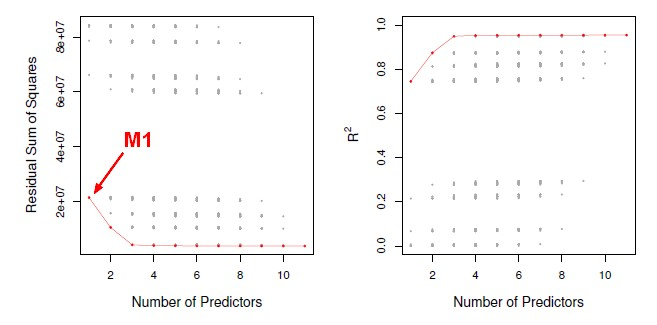# Statistics - Akaike information criterion (AIC)

AIC stands for Akaike Information Criterion.

Akaike is the name of the guy who came up with this idea.

AIC is a quantity that we can calculate for many different model types, not just linear models, but also classification model such logistic regression and so on.

## Definition

The AIC criterion is defi ned for a large class of models fi t by maximum likelihood:

$$AIC = -2 log L + 2 . d$$

where:

• L is the maximized value of the likelihood function for the estimated model.
• d is the total # of parameters used in the model (regression coefficients + intercept)

## Linear model

It turns out that in the case of a linear model with Gaussian errors, negative 2 log L is just equal to RSS over $\hat{\sigma}$ squared

$$2 log L = \frac{\href{RSS}{RSS}}{\href{variance}{\hat{\sigma}}^2}$$

where:

• $\hat{\sigma}^2$ is the variance estimate of the error associated with each response measurement (ie each error epsilon in the linear model)

Then by plugging it in the aboe formula, we can see that AIC and Mallow's Cp are actually proportional to each other. They are the same thing for linear models.

Recommended PagesData Mining - (Test|Expected|Generalization) Error

Test error is the prediction error that we incur on new data. The test error is actually how well we'll do on future data the model hasn't seen. The test error is the average error that results from using...Machine Learning - (Overfitting|Overtraining|Robust|Generalization) (Underfitting)

A learning algorithm is said to overfit if it is: more accurate in fitting known data (ie training data) (hindsight) but less accurate in predicting new data (ie test data) (foresight) Ie the model...A big R squared indicates a model that really fits the data well. But unfortunately, you can't compare models of different sizes by just taking the one with the biggest R squared because you can't compare...Statistics - Bayesian Information Criterion (BIC)

BIC is like AIC and Mallow's Cp, but it comes from a Bayesian argument. The formulas are very similar. The formula calculate the residual sum of squares and then add anadjustment term which is...Statistics - Model Selection

Model selection is the task of selecting a statistical model from a set of candidate models through the use of criteria's Dimension reduction procedures generates and returns a sequence of possible...Time Serie - Seasonality (Cycle detection)

Seasonality is a cycle in time serie. The season is used a discreet regression variable and code it as dummy variables). For instance: 1 if it's the season 0 if it's not the season If you...